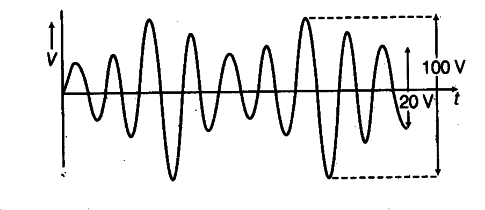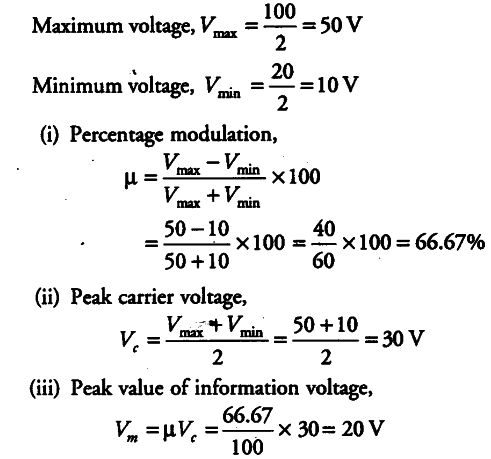# An amplitude modulated wave is as shown in the figure. Calculate

An amplitude modulated wave is as shown in the figure. Calculate
(i) the percentage modulation,
(ii) peak carrier voltage and
(iii) peak value of information voltage.From the figure,